#### 期刊菜单

Analysis of the Current Situation of Kuznets Curve in Fujian Province
DOI: 10.12677/SD.2020.103042, PDF, HTML, XML, 下载: 277  浏览: 1,206  科研立项经费支持

Abstract: Recently, the government departments pay more and more attention to environmental protection and ecological civilization construction, because the economic development and environmental pollution are always inseparable. According to Kuznets, the economist, the environmental quality will gradually deteriorate with the development of economy and then improve after reaching a turning point. This theory is called Kuznets curve theory. Based on this theory, this paper takes three indicators of environmental pollutants and economic development indicators in Fujian Province for example, and then uses Python language to analyze it. The research results show that the relationship between the pollutant emission index and per capita GDP in Fujian Province con-forms to the characteristics of the Environmental Kuznets curve, and the overall environmental situation is developing well.

1. 引言

2. 福建省经济与环境发展状况

2.1. 经济总量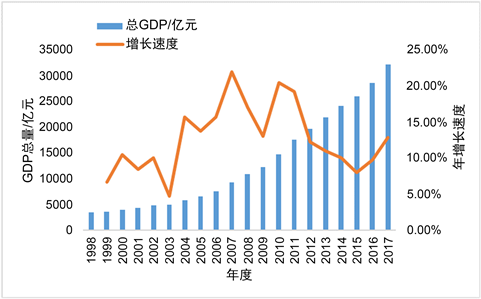Figure 1. Total GDP in Fujian Province from 1998 to 2017

2.2. 产业结构

1998年至2017年，福建省不断调整和优化产业结构。如图2，第一产业比重持续下降，2017年相比1998年下降至12.4%，第二产业比重呈现先升后降的趋势，第三产业比重在2014年之前呈现较为稳定的状态，在近三年却有快速增长的苗头，2017年占比达45.4%。通过上图不难发现，近年来，福建省突出发展服务业和高新技术产业，高新技术产业工业产值占工业总产值的比重不断提升，初步形成以服务业为主体、高新技术产业为主导的产业结构。为了持续推动高新技术产业的发展，研究人均GDP和环境污染之间的关系显得尤为重要。

2.3. 能源消费结构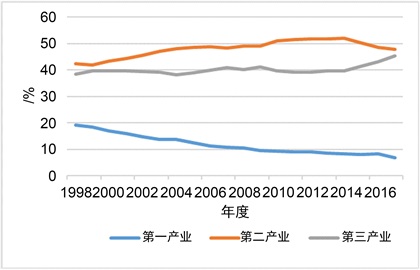Figure 2. Changes of industrial structure in Fujian province from 1998 to 2017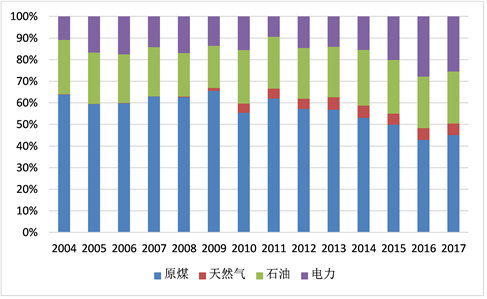Figure 3. Structure of industrial energy consumption from 2004 to 2017

3. 数据来源及研究方法

3.1. 数据来源Table 1. Economic environment indicators in Fujian Province from 1998 to 2017

3.2. 研究方法

${Y}_{1t}={\alpha }_{0}+{\alpha }_{1}{X}_{t}+{\alpha }_{2}{X}_{t}^{2}+{\delta }_{t}$ (1)

${Y}_{2t}={\beta }_{0}+{\beta }_{1}{X}_{t}+{\beta }_{2}{X}_{t}^{2}+{\delta }_{t}$ (2)

${Y}_{3t}={\chi }_{0}+{\chi }_{1}{X}_{t}+{\chi }_{2}{X}_{t}^{2}+{\delta }_{t}$ (3)

4. 福建省经济发展指标与环境污染方程拟合结果分析

4.1. 工业固体废物排放总量的方程拟合

$\text{GDP}=30869.713{T}_{0}+39524.3931{T}_{1}+14164.319{T}_{2}$ (4)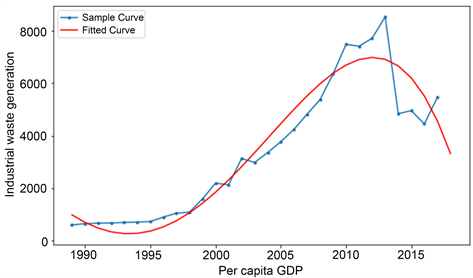Figure 4. Industrial waste generation

${Y}_{1t}=-3.048{e}^{-6}{x}^{2}+0.3041x-598.7$ (5)

${{Y}^{\prime }}_{1t}=-6.097{e}^{-6}x+0.3041$ (6)

4.2. 工业废气排放量的方程拟合

$GDP=49723.839{T}_{0}+66041.735{T}_{1}+21827.536{T}_{2}$ (7)

${Y}_{2t}=-2.423{e}^{-6}{x}^{2}+0.4308x-625$ (8)

${{Y}^{\prime }}_{2t}=-4.846{e}^{-6}x+0.4308$ (9)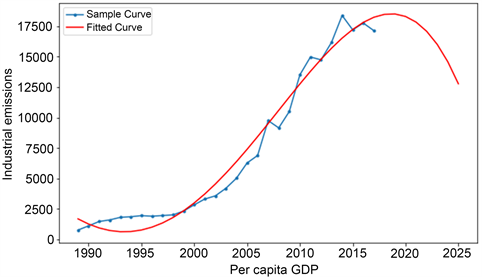Figure 5. Industrial emissions

4.3. 工业废水排放量的方程拟合

$\text{GDP}=33259.9994{T}_{0}+42908.3713{T}_{1}+15158.0108{T}_{2}$ (10)

${Y}_{3t}=-5.702{e}^{-5}{x}^{2}+4.629x+4.377{e}^{4}$ (11)

${{Y}^{\prime }}_{3t}=-0.000114\text{x}+4.629$ (12)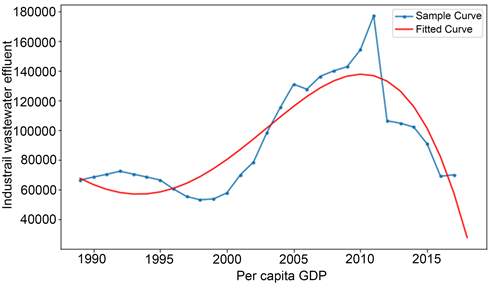Figure 6. Industrial wastewater effluent

5. 结论及建议

  KUZNETSS. (1995) Economic Growth and Income Inequality. The American Economic Review, No. 8, 1-28.  Panayoutou, T., Perrings, C.A., Molerk, G., et al. (1994) Conservation of Biodiversity and Economic De-velopment: The Concept of Transferable Development Right. Environmental and Resource Economics, 4, 91-110. https://doi.org/10.1007/BF00691934  张剑. 新旧动能转换背景下烟台市环境库兹涅茨曲线及影响因素研究[J]. 应用能源技术, 2019(8): 8-12.  尚杰, 李新, 邓雁云. 基于EKC的农业经济增长与农业面源污染的关系分析——以黑龙江省为例[J]. 生态经济, 2017, 33(6): 157-160 + 166.  李春梅. 环境库兹涅茨曲线之于北京市的适用性分析[J]. 城市问题, 2017(4): 34-40.  柯文岚, 沙景华, 闫晶晶. 山西省环境库兹涅茨曲线特征及其影响因素分析[J]. 中国人口•资源与环境, 2011, 21(S2): 389-392.  王懿. 安徽省环境库兹涅茨曲线现状分析[J]. 蚌埠学院学报, 2020, 9(1): 47-52.